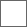• 0回复贴，共1

### 为什么Java中1000==1000为false而100==100为true

Integer a = 1000, b = 1000;
System.out.println(a == b);//1
Integer c = 100, d = 100;
System.out.println(c == d);//2你会得到false true

Integer c = 100;

Integer i = Integer.valueOf(100);

public static Integer valueOf(int i) {
if (i >= IntegerCache.low && i
return IntegerCache.cache[i + (-IntegerCache.low)];
return new Integer(i);
}

Integer c = 100, d = 100;

System.out.println(c == d);

public static void main(String[] args) throws NoSuchFieldException, IllegalAccessException {
Class cache = Integer.class.getDeclaredClasses(); //1
Field myCache = cache.getDeclaredField("cache"); //2
myCache.setAccessible(true);//3
Integer[] newCache = (Integer[]) myCache.get(cache); //4
newCache = newCache; //5
int a = 2;
int b = a + a;
System.out.printf("%d + %d = %d", a, a, b); //
}

#### 扫二维码下载贴吧客户端广告